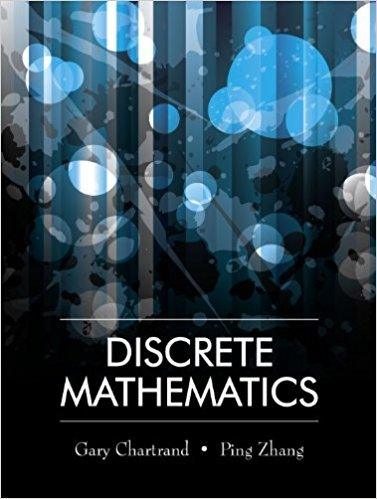×
×

# Solutions for Chapter 13.1: Fundamental Properties of Trees## Full solutions for Discrete Mathematics | 1st Edition

ISBN: 9781577667308Solutions for Chapter 13.1: Fundamental Properties of Trees

Solutions for Chapter 13.1
4 5 0 264 Reviews
22
4
##### ISBN: 9781577667308

Since 23 problems in chapter 13.1: Fundamental Properties of Trees have been answered, more than 13911 students have viewed full step-by-step solutions from this chapter. This expansive textbook survival guide covers the following chapters and their solutions. Discrete Mathematics was written by and is associated to the ISBN: 9781577667308. Chapter 13.1: Fundamental Properties of Trees includes 23 full step-by-step solutions. This textbook survival guide was created for the textbook: Discrete Mathematics, edition: 1.

Key Math Terms and definitions covered in this textbook
• Block matrix.

A matrix can be partitioned into matrix blocks, by cuts between rows and/or between columns. Block multiplication ofAB is allowed if the block shapes permit.

• Cayley-Hamilton Theorem.

peA) = det(A - AI) has peA) = zero matrix.

• Characteristic equation det(A - AI) = O.

The n roots are the eigenvalues of A.

• Eigenvalue A and eigenvector x.

Ax = AX with x#-O so det(A - AI) = o.

• Factorization

A = L U. If elimination takes A to U without row exchanges, then the lower triangular L with multipliers eij (and eii = 1) brings U back to A.

• Four Fundamental Subspaces C (A), N (A), C (AT), N (AT).

Use AT for complex A.

• Fourier matrix F.

Entries Fjk = e21Cijk/n give orthogonal columns FT F = nI. Then y = Fe is the (inverse) Discrete Fourier Transform Y j = L cke21Cijk/n.

• Full row rank r = m.

Independent rows, at least one solution to Ax = b, column space is all of Rm. Full rank means full column rank or full row rank.

• Gram-Schmidt orthogonalization A = QR.

Independent columns in A, orthonormal columns in Q. Each column q j of Q is a combination of the first j columns of A (and conversely, so R is upper triangular). Convention: diag(R) > o.

• Graph G.

Set of n nodes connected pairwise by m edges. A complete graph has all n(n - 1)/2 edges between nodes. A tree has only n - 1 edges and no closed loops.

• Hessenberg matrix H.

Triangular matrix with one extra nonzero adjacent diagonal.

• Jordan form 1 = M- 1 AM.

If A has s independent eigenvectors, its "generalized" eigenvector matrix M gives 1 = diag(lt, ... , 1s). The block his Akh +Nk where Nk has 1 's on diagonall. Each block has one eigenvalue Ak and one eigenvector.

• Lucas numbers

Ln = 2,J, 3, 4, ... satisfy Ln = L n- l +Ln- 2 = A1 +A~, with AI, A2 = (1 ± -/5)/2 from the Fibonacci matrix U~]' Compare Lo = 2 with Fo = O.

• Multiplier eij.

The pivot row j is multiplied by eij and subtracted from row i to eliminate the i, j entry: eij = (entry to eliminate) / (jth pivot).

• Row picture of Ax = b.

Each equation gives a plane in Rn; the planes intersect at x.

• Row space C (AT) = all combinations of rows of A.

Column vectors by convention.

• Schur complement S, D - C A -} B.

Appears in block elimination on [~ g ].

• Subspace S of V.

Any vector space inside V, including V and Z = {zero vector only}.

• Unitary matrix UH = U T = U-I.

Orthonormal columns (complex analog of Q).

• Volume of box.

The rows (or the columns) of A generate a box with volume I det(A) I.

×